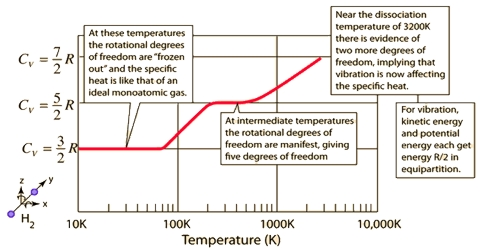Chemistry

# Heat Capacities of GasesHeat Capacities of Gases:

Heat capacity of a substance is defined as the heat necessary to raise the temperature of a given quantity of the substance through 10 C. Specific heat capacity or specific heat of a substance may be defined as the amount of heat necessary to raise the temperature of 1 g of the substance through 1°C. The specific heat of water at 15°C is just 1 calorie or 4.18 joule. If the temperature of a body is raised from T1 to T2 by adding an amount Q of heat, the heat capacity, C, of the body is given by:

C = Q / (T2 – T1)

Another quantity, molar heat capacity, is also defined as, the amount of heat necessary to raise the temperature of 1 mole of the substance through 10 C.

The value of the hest capacity will depend on whether heat has been added under constant pressure or constant volume conditions. This is particularly important in the case of gases as the heat capacity values at constant pressure (CP) and constant volume (CV) differ considerably.

This ratio changes with the atomicity of the gas. Its general equation is given below:

For any gas;

CP/CV = [5/2 R + x]/[ 3/2 R + x]

where X = 0, R and 3/2 R for mono-, di- and poly-atomic gases. By putting the value of X in the above equation, the ratio comes to 1.66, 1.40 and 1.33 for mono-, di- and poly-atomic gases, respectively.

The value indicates the atomicity of the gases; hereby atomic weight of the gas can be calculated as:

Atomic weight = Molecular weight/Atomicity